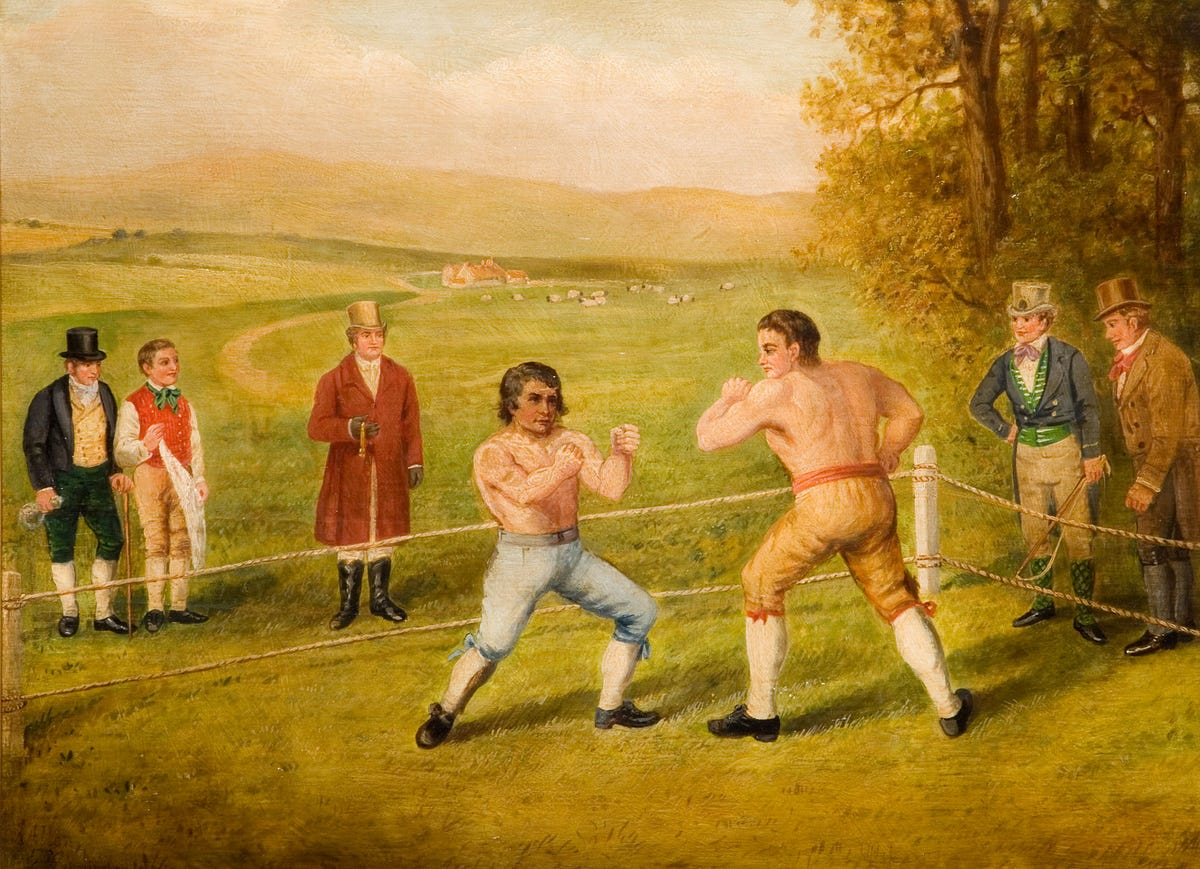# Gaussian Mixture Models vs K-Means. Which One to Choose?In this article, we will see that both models offer a different performance in terms of speed and robustness. We will also see that it is possible to use K-Means as an initializer for GMs which tends to boost the performance of the clustering model.

K-Means and Gaussian Mixtures (GMs) are both clustering models. Many data scientist, however, tend to choose a more popular K-Means algorithm. Even if GMs can prove superior in certain clustering problems.

In this article, we will see that both models offer a different performance in terms of speed and robustness. We will also see that it is possible to use K-Means as an initializer for GMs which tends to boost the performance of the clustering model.

## How They Work

First, let’s review the theoretical part of these algorithms. It will help us to understand their behaviour later in the article.

### K-Means

K-Means is a popular non-probabilistic clustering algorithm. The goal of the algorithm is to minimize the distortion measure J*. *We achieve that by the following iterative procedure :

1. Choose the number of clusters K
2. Initialize the vector μ_k that defines a central point of each cluster
3. Assign each data point *x *to the closest cluster centre
4. Recalculate central points *μ_k *foreach cluster
5. Repeat 3–4 until central points stop moving

## K-Means Clustering: How It Works & Finding The Optimum Number Of Clusters In The Data

K-Means Clustering: How It Works & Finding The Optimum Number Of Clusters In The Data. Mathematical formulation, Finding the optimum number of clusters and a working example in Python

## 15 Machine Learning and Data Science Project Ideas with Datasets

Learning is a new fun in the field of Machine Learning and Data Science. In this article, we’ll be discussing 15 machine learning and data science projects.

## Basics of Machine Learning: K-Means Clustering

Basics of Machine Learning: K-Means Clustering. As we dive into the world of “Unsupervised” Machine Learning, we will encounter problems that would require us to cluster the data available to us.

## Most popular Data Science and Machine Learning courses — July 2020

Most popular Data Science and Machine Learning courses — August 2020. This list was last updated in August 2020 — and will be updated regularly so as to keep it relevant

## “How’d you get started with machine learning and data science?”

“How’d you get started with machine learning and data science?”: I trained my first model in 2017 on my friend's lounge room floor.